## Numerical Simulation of Compressible Flows

Highlights and Challenges

Chalmers University of Technology
Department of Mechanics and Maritime Sciences
Division of Fluid Dynamics

https://nikander.github.io/Presentations/

Compressible flow is a branch of fluid mechanics that deals with flows having significant changes in fluid density

"Significant changes in fluid density"
- what does that mean?

$\class{MyRed}{\frac{\Delta \rho}{\rho}\gt 0.05}$
$\Delta p = c_{\infty}^2 \Delta \rho$ $SPL=20\log\left(\frac{p_{rms}}{p_{ref}}\right)=20\log\left(\frac{\Delta p}{\sqrt{2} p_{ref}}\right)$ $\left\{p=101325\ Pa,\ T=288\ K \Rightarrow \rho=1.23\ kg/m^3,\ c_\infty=340.17\ m/s \right\}$ $\class{MyBlue}{SPL=117\ dB}$
$\frac{\Delta \rho}{\rho}>0.05\Rightarrow M_\infty\gt\sqrt{\frac{0.1}{\gamma}}$ for air $$\left\{\gamma=1.4\right\}$$ $\class{MyBlue}{M_\infty\gt 0.27}$
• propagation of acoustics waves
• high-speed flows
• flows with large pressure variations• Related to the mean molecular velocity
• Changes with temperature $$\left(c=\sqrt{\gamma R T}\right)$$
• Inversely proportional to the fluid compressibility
• Finite Volume
• structured Meshes
• parallel (MPI/PETSc/SLEPc)
• Time-Marching technique
• explicit three-stage second-order Runge-Kutta
• dual time stepping/residual smoothing/low-speed preconditioning
• Convective fluxes
• third-order low-dissipation upwind scheme
Example:
• cube with the side $$L=1.0\ m$$
• resolve acoustic waves in the range $$f=50\ Hz\ -\ 10000\ Hz$$
• 20 cells per wavelength

Upper frequency limit $$\left(f=10000\ Hz\right)$$:

$$\lambda=c/f=0.034\ m\ \Rightarrow \Delta x < \lambda/20 = 0.0017\ m$$

Number of cells: $$(L/\Delta x)^3>{\class{MyRed}{200 \times 10^6}}$$

Space and time resolution:
• acoustic waves in large domains
• thin boundary layers
• shocks
• high relative velocities (turbomachinery)
• weak acoustic waves in high-energy flows
BOUNDARY CONDITIONS!
• acoustic reflections
• back flow
• extrapolation errors

### FLOW-INDUCED SOUND

Turbulence noise:
• Shear flows
• Boundary layers
• CombustionChallenges
• Numerical accuracy
• Mesh requirements
• Boundary conditions
Tonal Noise
• Rotor/stator interaction
• Feedback mechanisms
• Buzz-saw noiseChallenges
• Boundary conditions
• Number of harmonics
• Propagation of acoustics

### ACOUSTIC ANALOGIES

The analogy between the non-linear flow and the linear theory of acoustics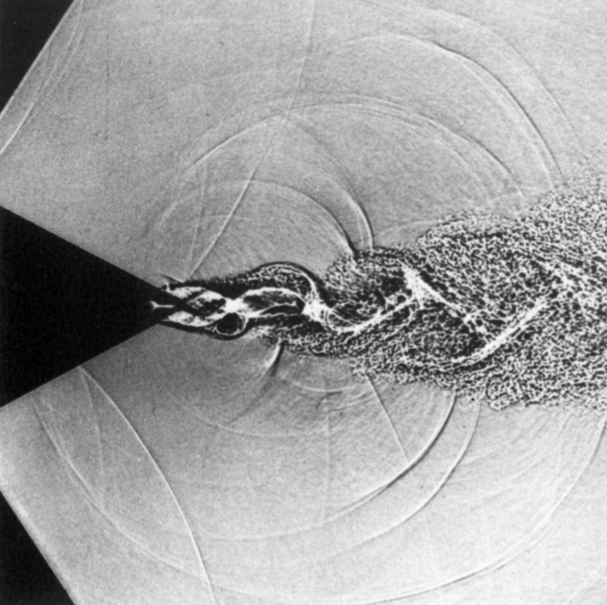A. Hirschberg, Introduction to aero-acoustics of internal flows, Advances in Aeroacoustics, VKI, 12-16 March 2001

Starting point:

continuity equation:

$\frac{\partial \rho}{\partial t}+\frac{\partial(\rho u_i)}{\partial x_i}=0$

momentum equation:

$\frac{\partial (\rho u_i)}{\partial t}+\frac{\partial (\rho u_i u_j)}{\partial x_j}=\frac{\partial }{\partial x_j}(\sigma_{ij}-p \delta_{ij})$

Step 1. the temporal derivative of the continuity equation

$\frac{\partial^2 \rho}{\partial t^2}+{\class{MyRed}{\frac{\partial^2(\rho u_i)}{\partial x_i \partial t}}}=0$

Step 2. the divergence of the momentum equation

${\class{MyRed}{\frac{\partial^2 (\rho u_i)}{\partial x_i \partial t}}}+\frac{\partial^2 (\rho u_i u_j)}{\partial x_i \partial x_j}=\frac{\partial^2 }{\partial x_i \partial x_j}(\sigma_{ij}-p \delta_{ij})$

Step 3. eliminate the highlighted terms

$\frac{\partial^2 \rho}{\partial t^2}=\frac{\partial^2 }{\partial x_i \partial x_j}(\rho u_i u_j - \sigma_{ij} + p \delta_{ij})$

Now, subtracting $${\class{MyBlue}{a_\infty^2\frac{\partial^2 \rho}{\partial x_i^2}}}$$ on each side gives us Lighthill's acoustic analogy

$\frac{\partial^2 \rho}{\partial t^2}-{\class{MyBlue}{a_\infty^2\frac{\partial^2 \rho}{\partial x_i^2}}}=\frac{\partial^2 T_{ij}}{\partial x_i \partial x_j}$

where $$T_{ij}$$ is the Lighthill stress tensor defined as

$T_{ij}=\rho u_i u_j - \sigma_{ij} + (p-{\class{MyBlue}{a_\infty^2\rho}}) \delta_{ij}$

($$a_\infty$$ is the speed of sound in an observer location)

Applying a free-space Green's function, Lighthill's equation can be solved analytically $\rho({\mathbf{y}},t)-\rho_\infty=\frac{1}{4 \pi a_\infty^2}\int_\Omega \frac{1}{r}\frac{\partial^2 T_{ij}}{\partial x_i \partial x_j}({\mathbf{x}},\tau_r)dV({\mathbf{x}})$
 $$\Omega$$ volume containing sound sources $${\mathbf{y}}$$ observer location $${\mathbf{x}}$$ source location $$r$$ distance between source and observer $$\tau_r$$ retarded time
• A Krylov subspace technique for extraction of global flow field modes
• Post processing technique applied to series of flow field snap shots
• Independent of data origin
• Possible to apply to truncated data sets

Assume linear mapping, $$B$$, of the flow dynamics

$Q^{(n+1)}=BQ^{(n)}$

where $$Q^n$$ is the flow field at time $$t^n$$ and $$Q^{n+1}$$ the flow field at time $$t^{n+1}=(t^n+\Delta t)$$

• $$Q^{(k)},\ k\in\{1, 2, \dots, n+2\}$$ is a set of flow-field vectors sampled with a specified frequency

• Differences of consecutive sampled solver states are used to define matrices $$V_n$$ and $$V_{n+1}$$
$V_n=\left\{Q^{(2)}-Q^{(1)},\dots,Q^{(n+1)}-Q^{(n)}\right\}$
$V_{n+1}=\left\{Q^{(3)}-Q^{(2)},\dots,Q^{(n+2)}-Q^{(n+1)}\right\}$

Using SVD, the matrix $$V_n$$ can be decomposed as

$V_n=U\Sigma W^*$

and since $$V_{n+1}=BV_n$$

$V_{n+1}=BV_n=B U\Sigma W^*$
 $$U$$ $$\left(m\times p\right)$$ $$\Sigma$$ $$\left(p\times p\right)$$ $$W^*$$ $$\left(p\times n\right)$$

Multiplying both sides by $$U^*$$ from the left

${\class{MyGreen}{U^*V_{n+1}}}=\underbrace{\class{MyRed}{U^*BU}}_{\class{MyBlue}{C}}{\class{MyGreen}{\Sigma W^*}}$

where $$\class{MyBlue}{C}$$ is the projection of the system matrix ($$B$$) on $$U$$

The projected system matrix can now be obtained without direct access to $$\class{MyRed}{B}$$ as

$\class{MyBlue}{C}={\class{MyGreen}{U^*V_{n+1}W\Sigma^{-1}}}$
• $$\class{MyBlue}{C}$$ is a $$\left(p\times p\right)$$ matrix, $$\left(m \gg n \ge p\right)$$

• The eigenvalues of $$\class{MyBlue}{C}$$ gives a good representation of the least damped eigenmodes of $$B$$

• Each eigenmode has a specific frequency

### SUPERSONIC JET AOCOUSTICS

Haukur Hafsteinsson & Markus Olander-Burak
FMV (SIGMUND)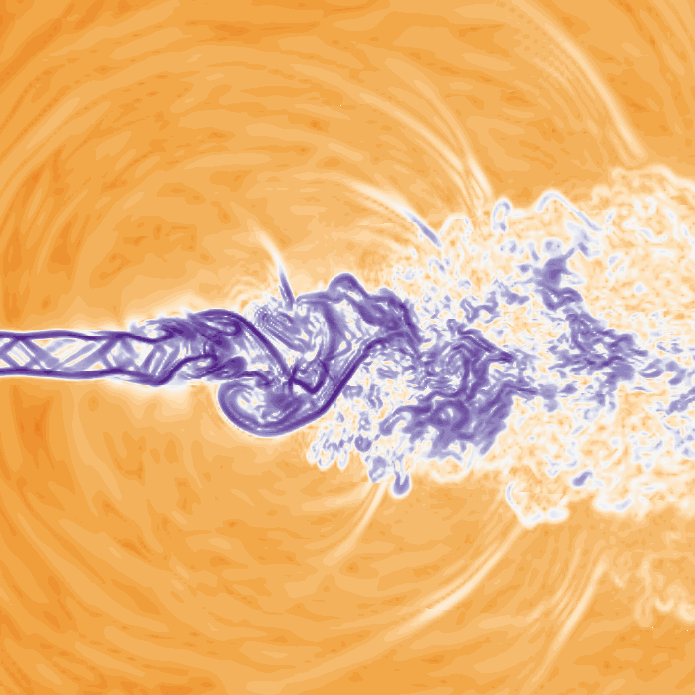Screech tone cancellation using fluid injection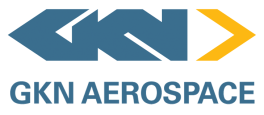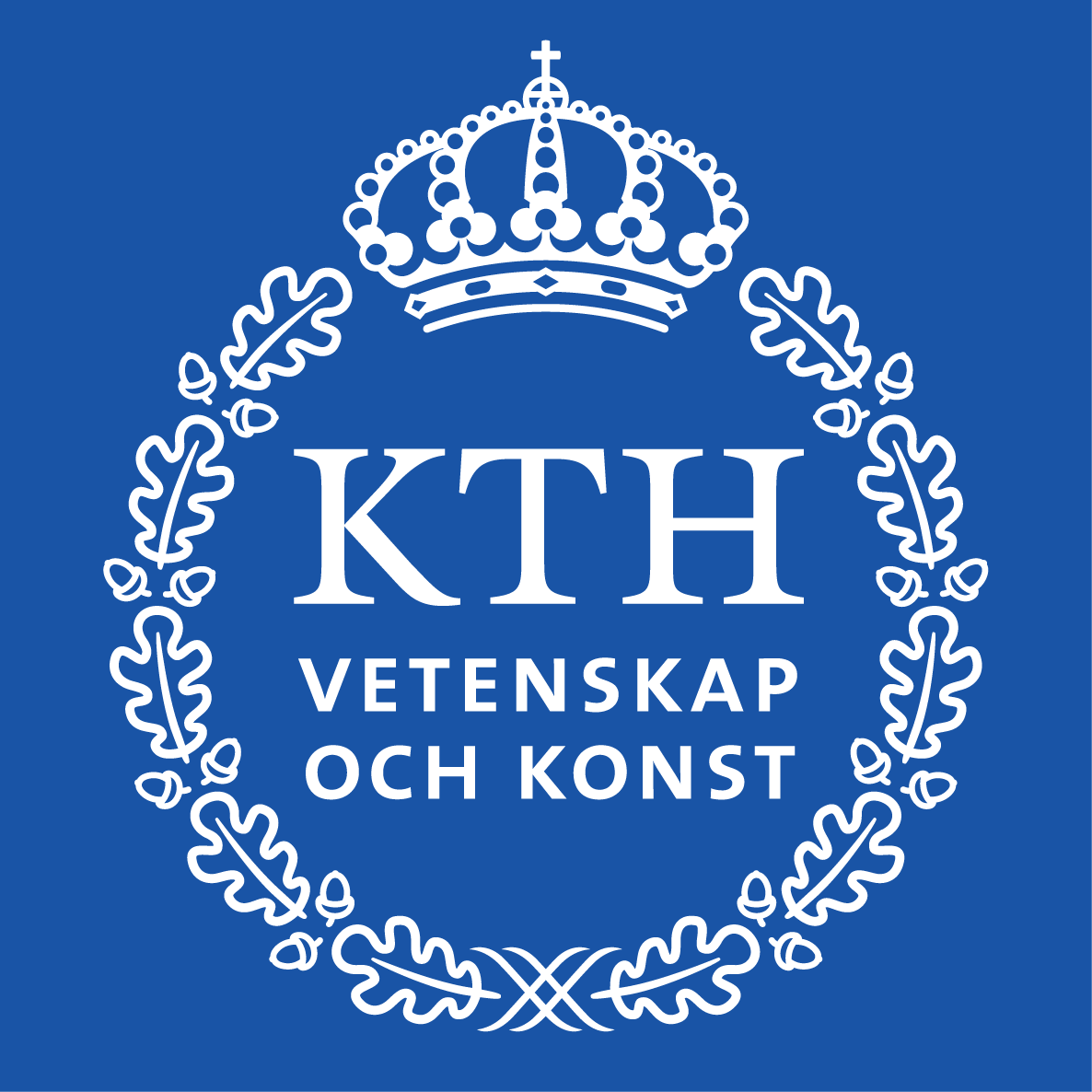Method:
• Compressible LES
• Ffowcs William-Hawking surface integration
• Dynamic Mode Decomposition (DMD)
Main challenges:
• Boundary conditions
• Short time step
• Noisy data• Feedback mechanism:
• flow structures at the nozzle lip
• upstream-traveling disturbancesHelical mode with the screech frequency detected using Dynamic Mode Decomposition (DMD)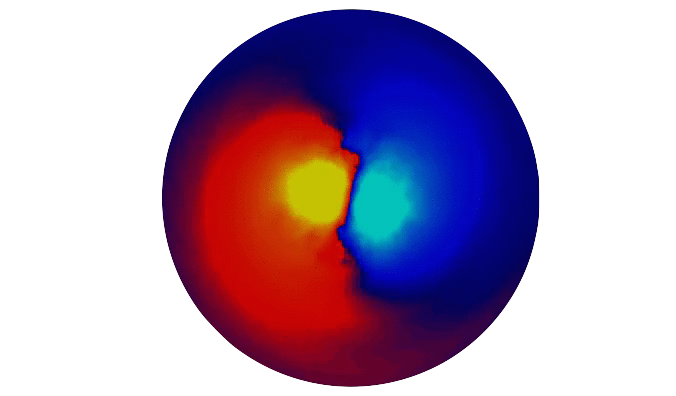Ragnar Lárusson
Swedish National Space Board (NRFP)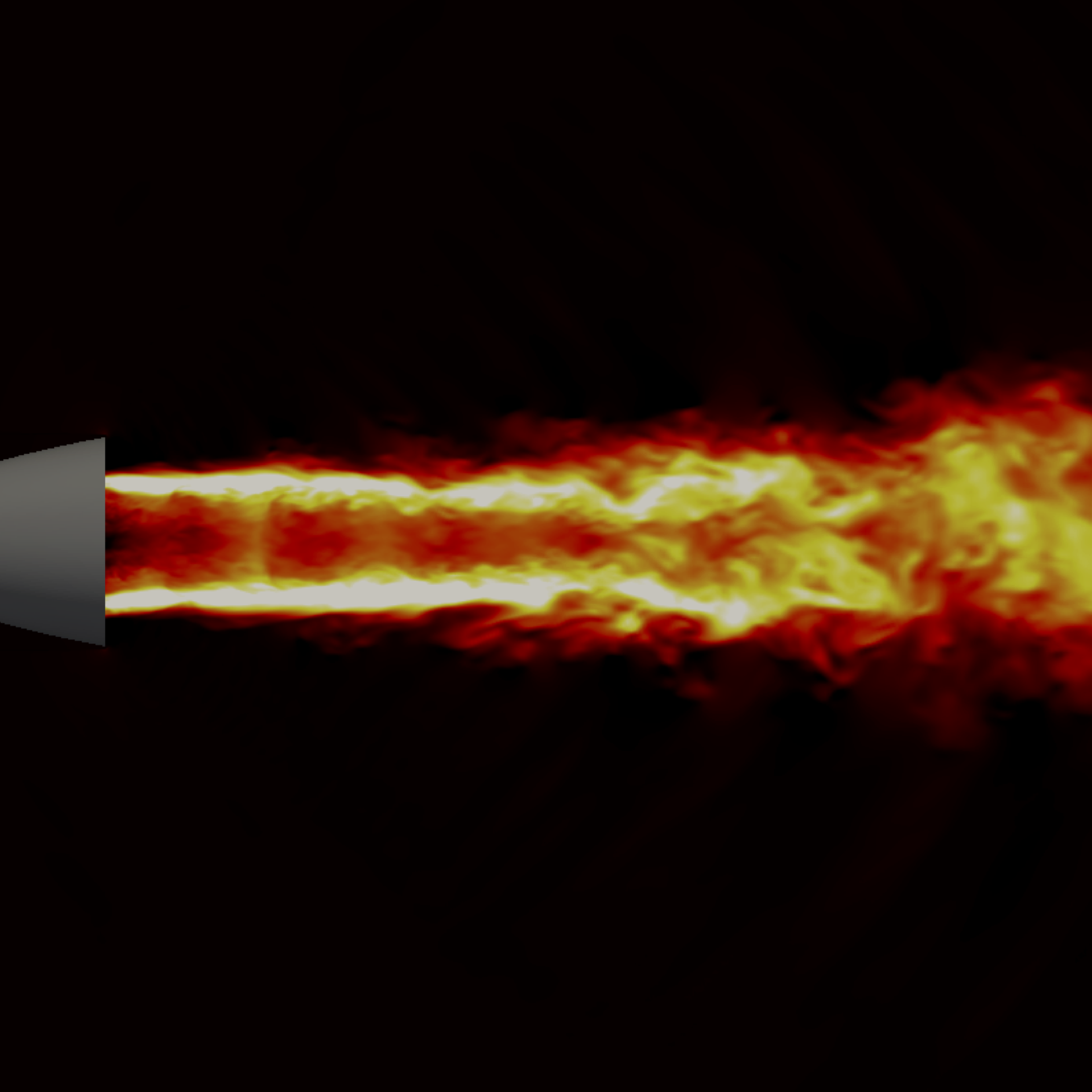Prediction of side loads during a space nozzle start-up sequenceMethod:
• Compressible URANS/DDES
• Dynamic Mode Decomposition
Main challenges:
• Short time step
• Noisy data• Simulated side-loads were lower than measured side-loads but within uncertainty levels
• DMD Identified modes responsible for side-loads and nozzle ovalization

### COMPRESSOR DUCT AREODYNAMICS

Vinnova (NFFP) & Clean Sky 2 (IDA)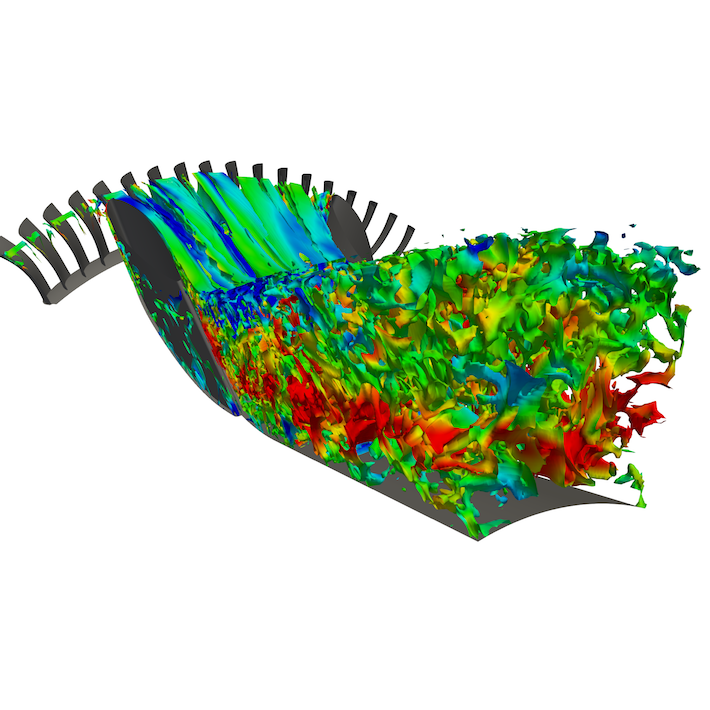Investigation of the aerodynamics of an intermediated compressor duct with integrated bleed systemMethod:
• Compressible DDES
• Spalart-Allmaras
Main challenges:
• Short time step
• Bleed tube boundary conditionsCode development
• Spalart-Allmaras DDES model implemented
• Significantly improved time-stepping technique
• dual time stepping
• five-stage Runge-Kutta
• implicit residual smoothing
• low-speed preconditioning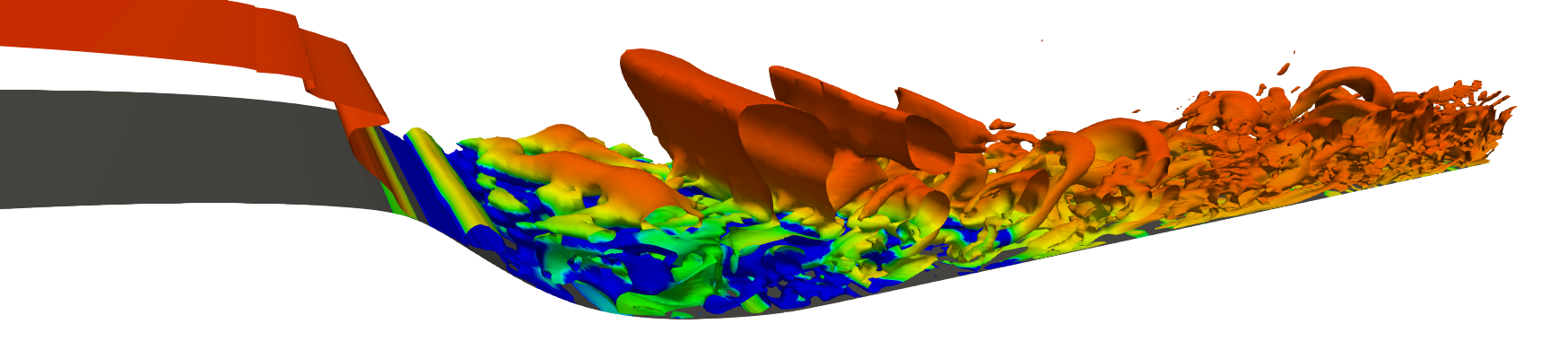Gonzalo Montero Villar
H2020 (TurboNoiseBB)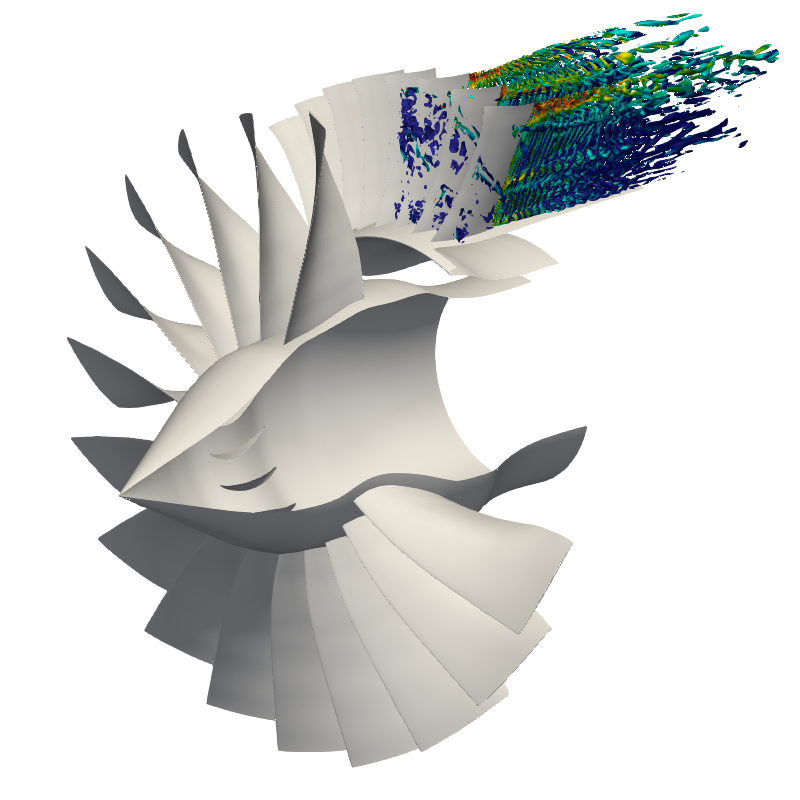Prediction of fan outlet guide vane broadband noise using detailed numerical methodsMethod:
• Compressible DDES/URANS
• Chorocronic tangential boundaries
• Specified wake-data from upstream rotor
• Inlet synthetic turbulence
• Ffowcs William & Hawking surface integration
Challenges:
• Very short time step
• Tangential boundary feedback loop
• Inlet turbulence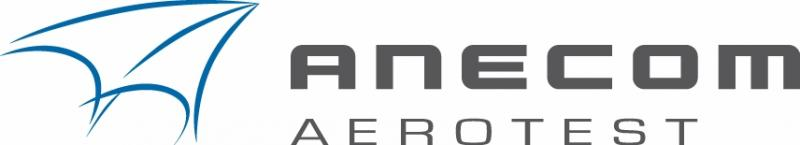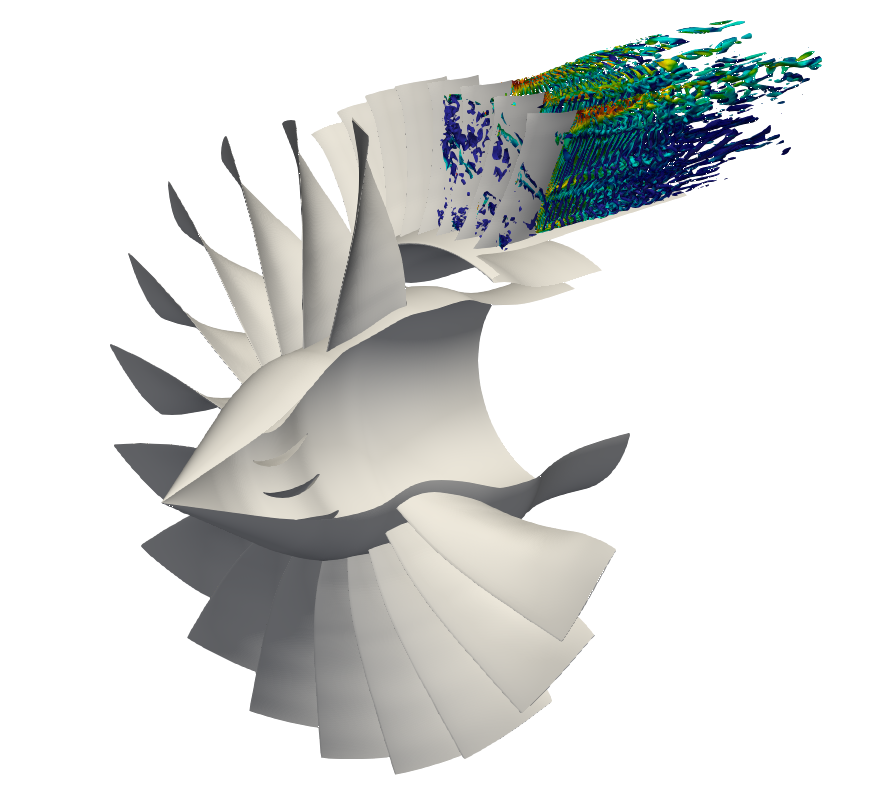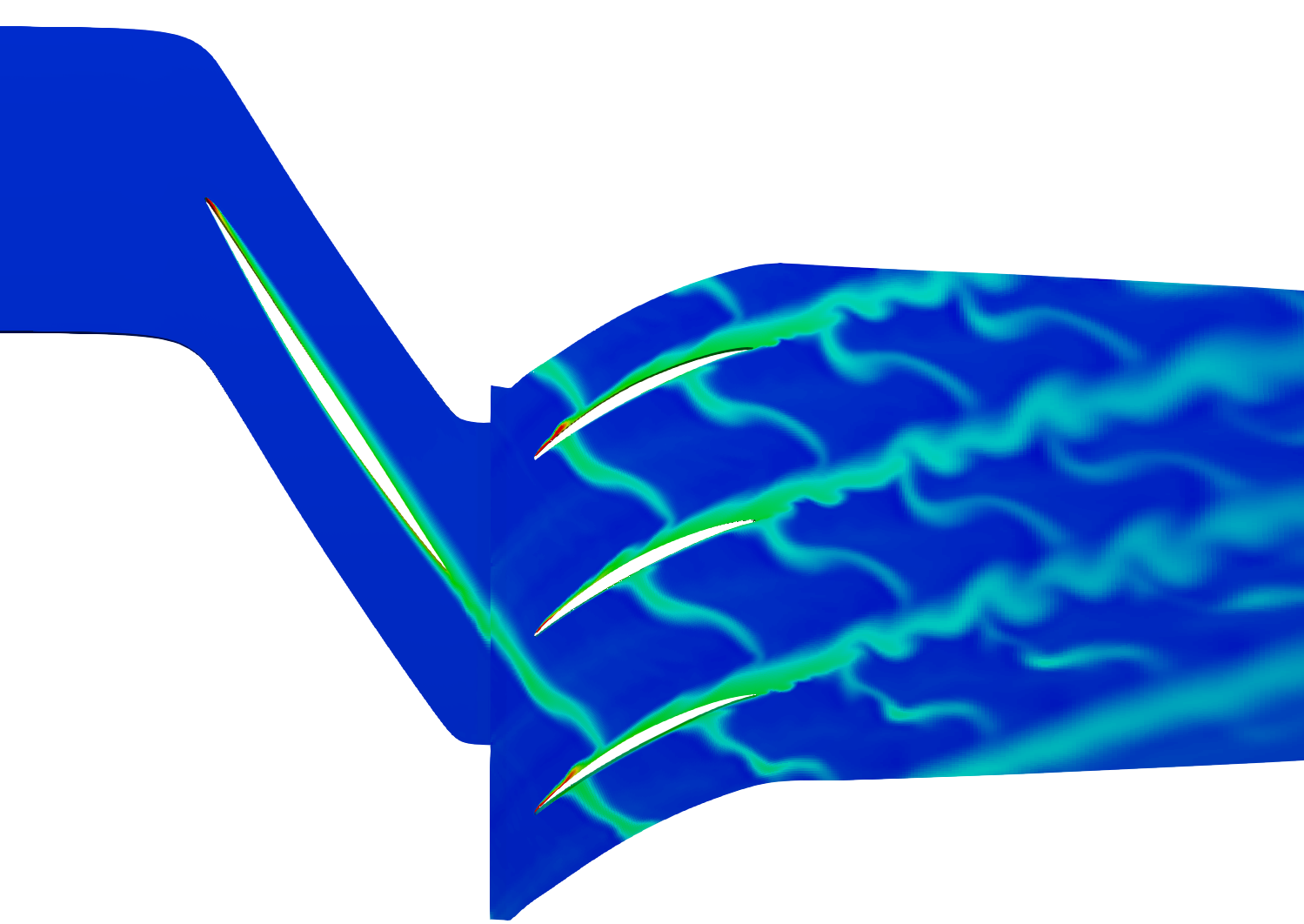### TURBOMACHINERY TONAL NOISE PREDICTION

H2020 (ULTIMATE)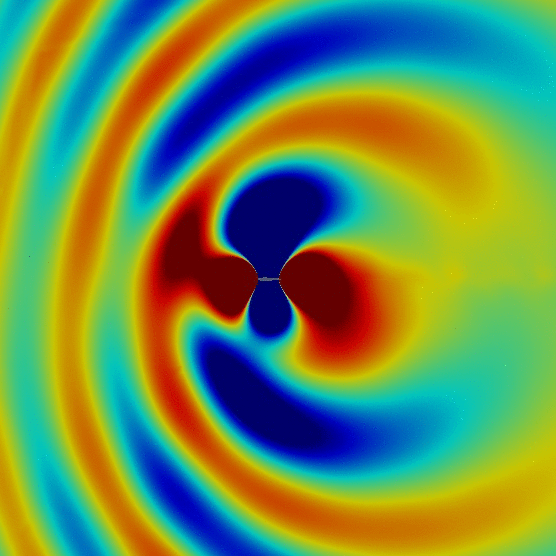Prediction of tonal noise of open rotorsMethod:
• Harmonic Balance
• Non-reflective boundary conditions
• Non-reflective rotor/stator interface
• Ffowcs William & Hawking surface integration (convective formulation)
Challenges:
• Boundary conditions
• Number of harmonics needed

A periodic solution can be represented by an infinite series of harmonics

$Q(t)=\sum_{n=-\infty}^{\infty}\hat{Q}_n e^{i\omega_n t}$

Truncating the series, we can get an approximation of the periodic solution

$Q(t)\approx\sum_{n=-N_h}^{N_h}{\hat{Q}}_n e^{i\omega_n t}$

Nyquist sampling theorem:

A solution containing $$N_h$$ harmonics is uniquely determined from its values at $$N_t=\left(2N_h+1\right)$$ samples uniformly distributed over one period

$\hat{Q}_n=\frac{1}{N_t}\sum_{l=0}^{N_t-1}Q_l e^{-i\omega_n t_l}$

### SOLVER ACCELERATION USING DMD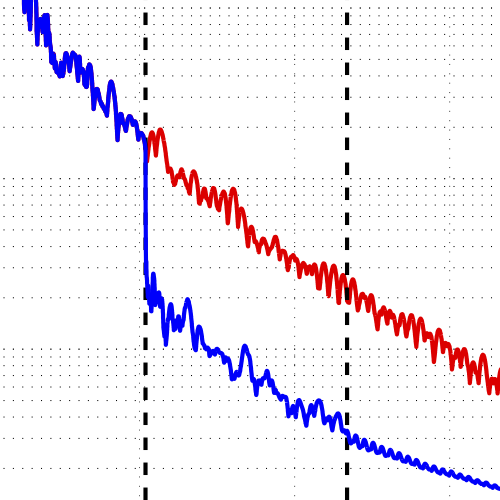Assume that a problem has a true steady-state solution, could the global mode information provided by DMD be used to find the steady-state condition?

$Q^{(n+1)}=Q^{(n)}=Q$

and thus the linear relation

$Q^{(n+1)}=BQ^{(n)}+b$

reduces to

$(I-B)Q=b$

Introducing a correction $$\Delta Q$$ that is obtained by subtracting sample $$Q^{(n+1)}$$ from $$Q$$, we get

$(I-B)\Delta Q=\underbrace{(B-I)Q^{(n+1)}+b}_{\class{MyGreen}{Q^{(n+2)}-Q^{(n+1)}=D}}$

where the right hand side corresponds to the last vector in $$V_{n+1}$$

Introduce $$\Delta q$$ defined by $$\Delta Q=U\Delta q$$

$(I-B)\Delta Q=(I-B)U\Delta q={\class{MyGreen}{D}}$

Multiplying both sides with $$U^*$$ from the left

${\class{MyRed}{U^*}}(I-{\class{MyRed}{B}}){\class{MyRed}{U}}\Delta q= \left\{ \class{MyBlue}{C}=\class{MyRed}{U^*BU},\ U^*U=I \right\}= (I-{\class{MyBlue}{C}})\Delta q = U^*{\class{MyGreen}{D}}$ $\Rightarrow \Delta q = (I-{\class{MyBlue}{C}})^{-1}U^*{\class{MyGreen}{D}}$

From the definition of $$\Delta Q$$ we get

$Q=Q^{(n+1)}+\Delta Q=Q^{(n+1)}+U\Delta q$
$Q=Q^{(n+1)}+U(I-{\class{MyBlue}{C}})^{-1}U^*{\class{MyGreen}{D}}$

where

${\class{MyBlue}{C}}=U^*V_{n+1}W\Sigma^{-1}$

Low-Mach-number ($$M<0.08$$) turbine cascade flow (2D)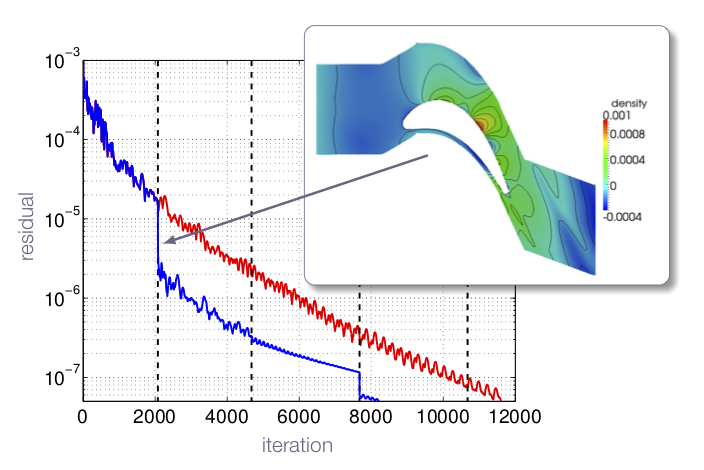### STIRLING ENGINE

Martin Nilsson
Vinnova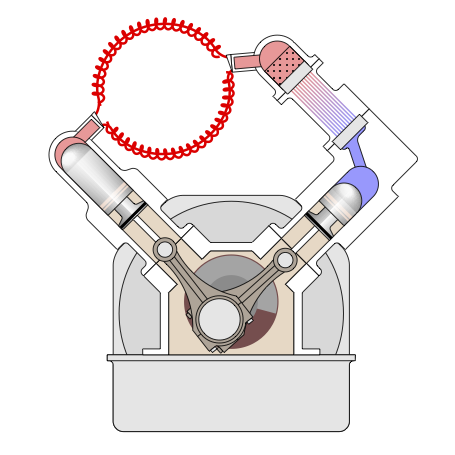Stirling engine optimizationMethod:
• Quasi-1D approach
• Modelling of heat transfer and flow losses
• Optimization
Challenges:
• Regenerator model
• Real gas effects
• Quasi-1D solver for simulating the flow in a Stirling engine developed
• Model validated against measured data
• Optimization framework setup and tested

Marcus Lejon & Gonzalo Montero Villar
Vinnova (NFFP)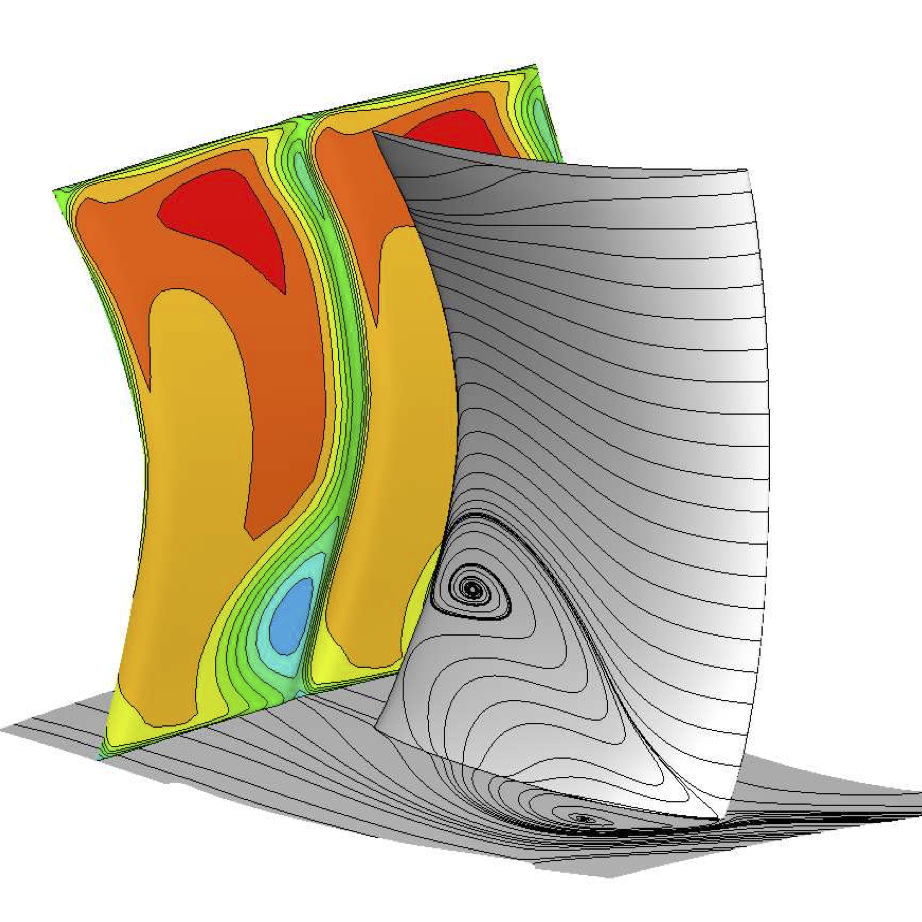Robust multi-objective optimization for compressor blade designMethod:
• Compressible RANS/URANS
• Multi-objective optimization
• Genetic algorithm
Challenges:
• Rotor tip-clearance
• Surge-margin predictionHAMON

### ACOUSTIC RADIATION IN URBAN AREAS

Guglielmo Minelli
Area of Advance Energy - Sustainable Vehicle Technology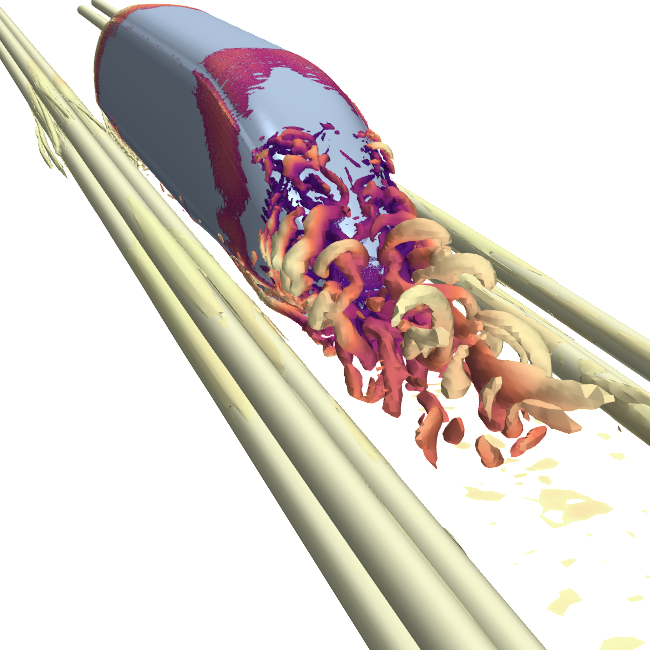Evaluation of noise canceling barriers for trains in urban areas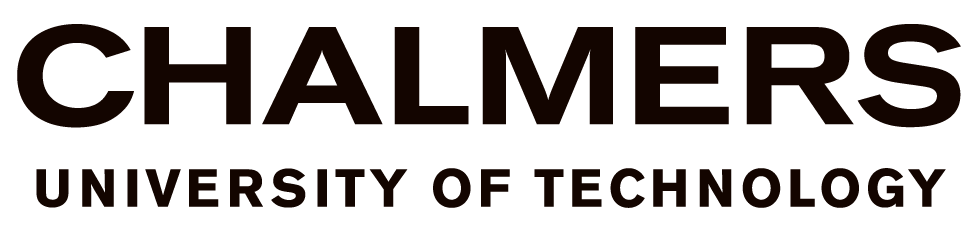Method:
• Compressible DDES
• Ffowcs William & Hawking surface integration
Challenges:
• Spatial resolution
• Tonal noise source identification### COMBUSTION INSTABILITIES

Swedish Energy Agency (HIGH2)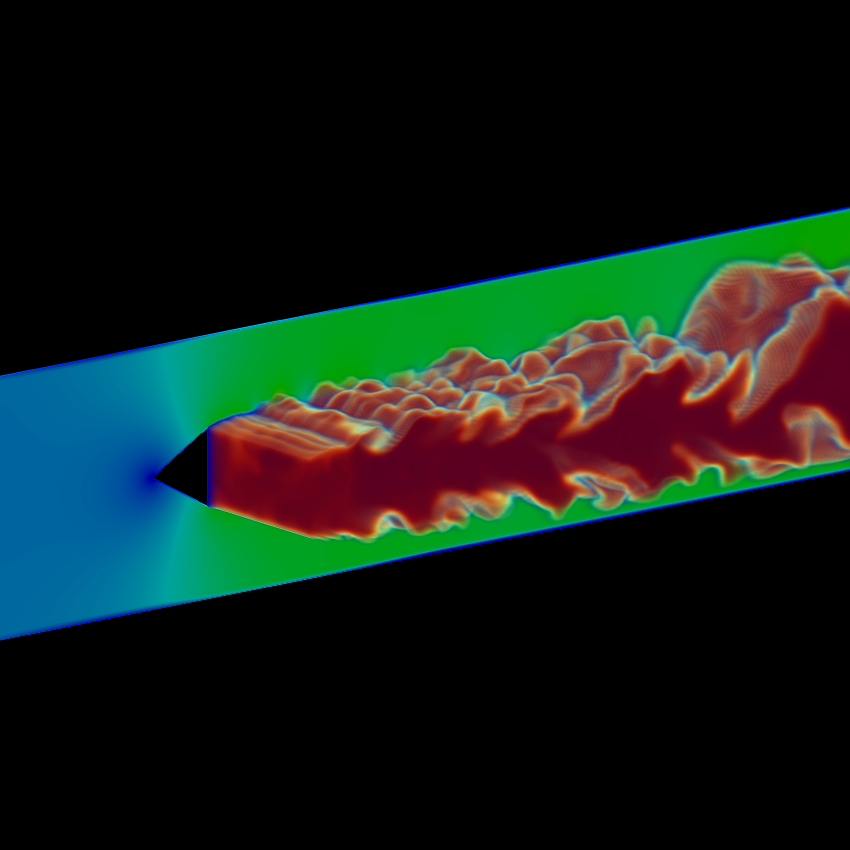Prediction of combustion instabilities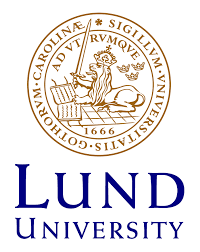Method:
• Compressible LES (Fluent)
Challenges:
• Combustion modelling
• Boundary conditions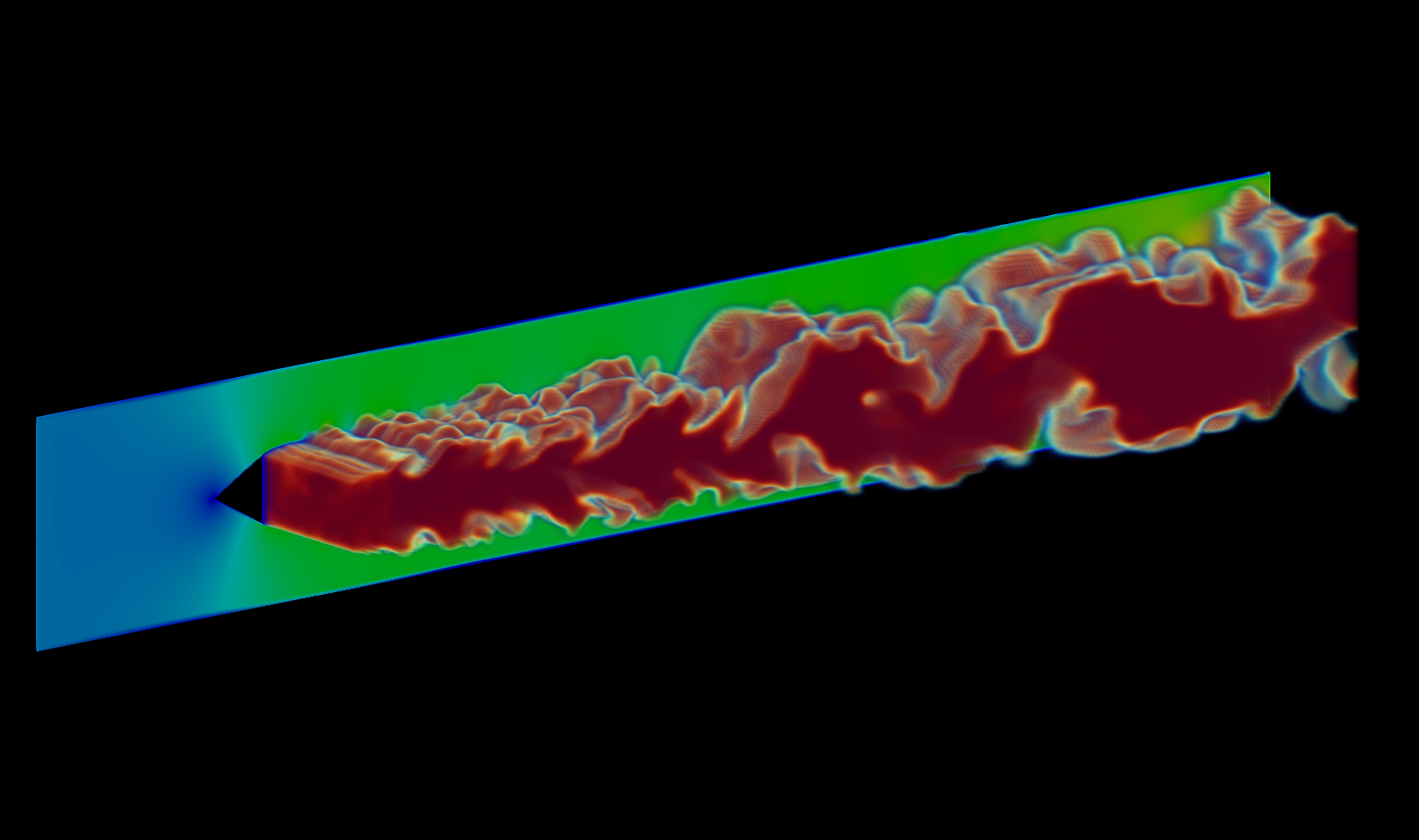past
• Prediction of sound generation and radiation
ongoing
• Analyzing noise-generating flow mechanisms
future
• Low-noise design (optimization)
• geometric definition
• integrated acoustic liners
past
• Modular design of isolated components
ongoing
• Detailed analysis of larger engine modules
• Engine module optimization
future
• Integrated design of engine components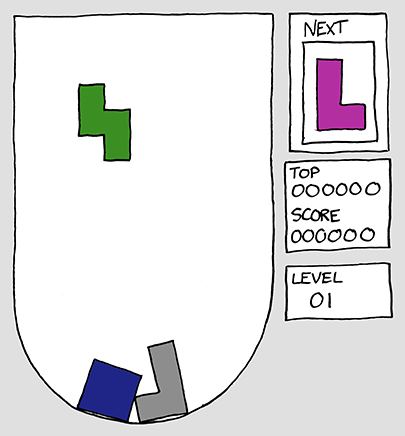https://xkcd.com/724/